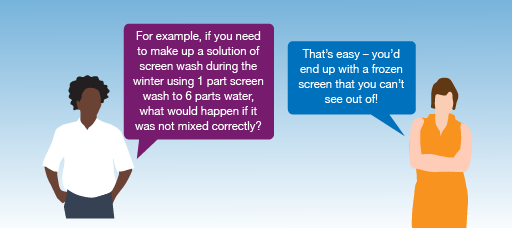Everyday maths for Construction and Engineering 1

Start this free course now. Just create an account and sign in. Enrol and complete the course for a free statement of participation or digital badge if available.

Free course

# 7 Ratios

Along with proportion (which you’ll look at in the next section), you use ratio in everyday activities such as mixing cement, painting your house and checking structural systems for safety issues, for example a roof.Figure 26 Talking ratios

Ratio is where one number is a multiple of the other. To find out more about ratios, read the following example.

## Example: How to use ratios

Suppose you need to make up one litre (1,000 ml) of bleach solution. The label says that to create a solution you need to add one part bleach to four parts water.

This is a ratio of 1 to 4, or 1:4. This means that the total solution will be made up of:

One part + four parts = five parts

If we need 1,000 ml of solution, this means that one part is:

1,000 ml ÷ 5 = 200 ml

The solution needs to be made up as follows:

Bleach: one part × 200 ml = 200 ml

Water: four parts × 200 ml = 800 ml

So to make one litre (1,000 ml) of solution, you will need to add 200 ml of bleach to 800 ml of water.

You can check your answer by adding the two amounts together. They should equal the total amount needed:

200 ml + 800 ml = 1,000 ml

Hint: m3 = cubic metres (m × m × m). You will look at volume later in the course.

## Activity 18: Using ratios

1. The ratio of sand to cement required to make concrete is 3:1.

How much of each is needed in order to make 60 m3 of concrete?

2. Read the label from a bottle of wallpaper stripper:

• Dilute: add 1 part wallpaper stripper to 7 parts water.

How much wallpaper stripper and water is needed to make 16 litres of solution?

3. To make a solution of screen wash you need to add one part of screen wash to six parts of water. How much screen wash and water is needed to make 700 ml of solution?

1. A ratio of 3:1 means three parts of sand to one part of cement, making four parts in total.

We need 60 m3 of concrete. If four parts are worth 60 m3, this means that one part is worth:

• 60 m3 ÷ 4 = 15 m3

So 60 m3 of concrete requires:

• Sand: three parts × 15 m3 = 45 m3

Cement: one part × 15 m3 = 15 m3

You can confirm that these figures are correct by adding them and checking that they match the amount needed:

• 45 m3 + 15 m3 = 60 m3
2. A ratio of 1:7 means one part of wallpaper stripper to seven parts of water, making eight parts in total.

We need 16 litres of solution. If eight parts are worth 16 litres, this means that one part is worth:

• 16 litres ÷ 8 = 2 litres

So 16 litres of solution requires:

• Wallpaper stripper: one part × 2 litres = 2 litres

Water: seven parts × 2 litres = 14 litres

You can confirm that these figures are correct by adding them and checking that they match the amount needed:

• 2 litres + 14 litres = 16 litres
3. The ratio of 1:6 means one part screen wash to six parts water, making seven parts in total.

We need 700 ml of solution. If seven parts are worth 700 ml, this means that one part is worth:

• 700 ml ÷ 7 = 100 ml

So 700 ml of solution requires:

• Screen wash: one part × 100 ml = 100 ml

Water: six parts × 100 ml = 600 ml

You can confirm that these figures are correct by adding them and checking that they match the amount needed:

• 100 ml + 600 ml = 700 ml

## Summary

You have now learned how to use ratio to solve problems in everyday life. This could be when you are mixing concrete, wallpaper stripper or screen wash. Can you think of any more examples where you might need to use ratio?

FSM_SSC_1